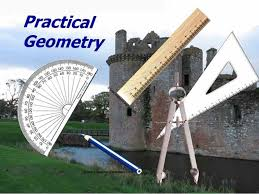# Practical Geometry: Construction of Geometrical Objects

In this quiz, you will be going to solve questions based on practical geometry, i.e construction of different geometrical objects like parallel lines, right-angled triangles, etc.Start Quiz

Identify the criterion of construction of the equilateral triangle LMN given LM = 6 cm.

S.A.S. criterion R.H.S. criterion A.S.A. criterion S.S.S. criterion

A Given AB = 3 cm, AC = 5 cm,and ∠B = 30°, ΔABC cannot be uniquely constructed, with AC as base, why?

Two sides and included angle are given. The other two angles are not given. The vertex B cannot be uniquely located. The vertex A coincides with the vertex C.

A line panda point X not on it are given. Which of the following is used to draw a line parallel to p through X?

Equal corresponding angles. Congruent triangles. Angle sum property of triangles. Pythagoras’ theorem.

In ΔRST, R = 5 cm, and ∠SRT = 45° and ∠RST = 45°. Which criterion can be used to construct ΔRST?

A.S.A. criterion S.A.S. criterion S.S.S. criterion R.H.S. criterion

Identify the false statement.

A triangle with three equal sides is called an equilateral triangle. A triangle with a right angle is called a right angled triangle. A triangle with two equal sides is called a scalene triangle. A right angled triangle has two acute angles and a right angle.

Which among the following is used to construct a triangle?

The lengths of the three sides. The perimeter of the triangle. The measures of three angles. The names of three vertices.

In which of the following cases is the construction of a triangle not possible?

Measures of 3 sides are given. Measures of 2 sides and an included angle are given. Measures of 2 angles and a side are given. Measures of 3 angles are given.

ΔPQR is a triangle right-angled at P. If PQ = 3 cm and PR = 4 cm, find QR.

3 cm 7 cm 5 cm 8 cm

The sum of the lengths of any two sides of a triangle is _____________ the third side of the triangle.

Less than

Doubled

Greater than

Half

Identify the true statement.

A triangle with 3 equal sides is isosceles.

A triangle with a 110o angle is right angled.

A triangle with 3 acute angles is acute angled.

A triangle with 2 equal sides is equilateral.

Quiz/Test Summary
Title: Practical Geometry: Construction of Geometrical Objects
Questions: 10
Contributed by: